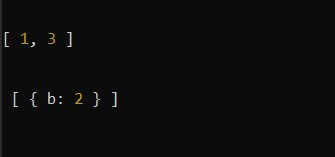# Lodash _.differenceWith() Method

Lodash _.differenceWith() method is similar to the _.difference() method that returns the array containing the values that are in the first array not in the second array but in _.differenceWith() all the elements of the first array are compared with the second array by applying comparison provided in third. It may be a little complex to understand by reading this but it will become simple when you see the example.

### Syntax:

`_.differenceWith(array, [values], [comparator]);`

### Parameters:

• array: This parameter holds the array that values are checked or inspected.
• values: This parameter holds the value that needs to be removed.
• comparator: This parameter holds the comparison invoked per element.

### Return Value:

This method returns an array according to the condition explained above.

Example 1: In this example, we are finding the difference of the arrays by using the lodash _.differenceWith() method.

## javascript

 `const _ = require(``'lodash'``)`   `let x = [1, 2, 3]` `let y = [2, 4, 5]`   `let result = _.differenceWith(x, y, _.isEqual);` `console.log(result);`

Output:

`[1, 3]`

Example 2: In this example, we are finding the difference of the arrays by using the lodash _.differenceWith() method.

## javascript

 `const _ = require(``'lodash'``);`   `let x = [{ a: 1 }, { b: 2 }, 6]` `let y = [{ a: 1 }, 7, 6]`   `let result = _.differenceWith(x, y, _.isEqual);` `console.log(result);`

Output:

`[{b: 2}]`

Example 3: In this example, we are finding the difference of the arrays by using the lodash _.differenceWith() method.

## javascript

 `const _ = require(``'lodash'``);`   `let x1 = [1, 2, 3]` `let y1 = [2, 4, 5]` `let result1 = _.differenceWith(x1, y1, _.isEqual);` `console.log(result1);`   `let x2 = [{ a: 1 }, { b: 2 }, 6]` `let y2 = [{ a: 1 }, 7, 6]` `let result2 = _.differenceWith(x2, y2, _.isEqual);` `console.log(result2);`

Output:Note: This will not work in normal JavaScript because it requires the library lodash to be installed.

Whether you're preparing for your first job interview or aiming to upskill in this ever-evolving tech landscape, GeeksforGeeks Courses are your key to success. We provide top-quality content at affordable prices, all geared towards accelerating your growth in a time-bound manner. Join the millions we've already empowered, and we're here to do the same for you. Don't miss out - check it out now!

Previous
Next